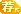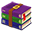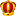##帐号 自动登录 找回密码 密码 注册[Register]
 搜索 热搜: ctf 新手 脱壳 教程

# [Android 原创] 2020年春节红包第四题wp[复制链接]发表于 2020-2-10 03:03

# 准备工具

IDA Pro，GDA，能真机调试的Android机一台

# GDA分析# IDA分析调试``````static main(void)
{
do
{
step_over();
wait_for_next_event(STEP,-1);
}while(PC!=0xEEDAFC2C);//停在何处
}``````IDA停在checkSn后，打开function tracing，跑一次脚本，等停在checkSnretn，按esc后退，找到跑飞处，在下面那句代码下断。清除trace记录后，在checkSn函数头再跑一次。# 分析checkSn的函数调用R6寄存器存放着GetStringUTFLength函数的地址，说明这里确实是取字符串长度的，至于是uid还pwd用排除法即可。pwd长度不足15，所以直接返回了，我们修改下假码，继续抓取，逐个分析即可。# 分析check函数

check函数首先调用gettimeofday，然后将timeval除以1800000，也就是30分钟。check函数的流程就走完了。

# 编写注册机

``````import hashlib
from Crypto.Cipher import ARC4
b64table = 'AzSxleoQp02MtvisIZUF8ThRaEL9Nd57qG6DfOkW4JHXmYjwV1Pn3uycrCgbKB-_='
decryptkey = '52pojie2020xtian'

def RC4(data, key):
rc41 = ARC4.new(key)
encrypted = rc41.encrypt(data)
return encrypted

def b64encode(s):
res = []
leftover = len(s) % 3
for i in range(0, len(s) - leftover, 3):
c2 = ord(s[i])
c1 = ord(s[i + 1])
c0 = ord(s[i + 2])
res.append(b64table[(c2 >> 2) & 0x3f])
res.append(b64table[((c2 & 0x3) << 4) | ((c1 >> 4) & 0x0f)])
res.append(b64table[((c1 & 0x0f) << 2) | ((c0 >> 6) & 0x03)])
res.append(b64table[c0 & 0x3f])
i += 3
if leftover == 1:
c2 = ord(s[i])
res.append(b64table[(c2 >> 2) & 0x3f])
res.append(b64table[(c2 & 0x3) << 4])
res.append(b64table[-1])
res.append(b64table[-1])
elif leftover == 2:
c2 = ord(s[i])
c1 = ord(s[i + 1])
res.append(b64table[(c2 >> 2) & 0x3f])
res.append(b64table[((c2 & 0x3) << 4) | ((c1 >> 4) & 0x0f)])
res.append(b64table[(c1 & 0x0f) << 2])
res.append(b64table[-1])
return ''.join(res)

def b64decode(s):
res = []
end = len(s)
if s[-1] == b64table[-1]:
end -= 4
for i in range(0, end, 4):
c3, c2, c1, c0 = b64table.index(s[i]), b64table.index(
s[i + 1]), b64table.index(s[i + 2]), b64table.index(s[i + 3])
res.append(chr(((c3 << 2)) | ((c2 >> 4) & 0x03)))
res.append(chr(((c2 & 0x0f) << 4) | ((c1 >> 2) & 0x0f)))
res.append(chr(((c1 & 0x03) << 6) | (c0 & 0x03f)))
if end < len(s):
if s[-2] == b64table[-1]:
c3, c2 = b64table.index(s[end]), b64table.index(s[end + 1])
res.append(chr(((c3 << 2)) | ((c2 >> 4) & 0x03)))
else:
c3, c2, c1 = b64table.index(s[end]), b64table.index(s[end + 1]), b64table.index(s[end + 2])
res.append(chr(((c3 << 2)) | ((c2 >> 4) & 0x03)))
res.append(chr(((c2 & 0x0f) << 4) | ((c1 >> 2) & 0x0f)))
return ''.join(res)

def wuaiencrypt(message):
r = ''
for i in list(message):
r += chr(ord(i) ^ 0x20)
r = RC4(r, decryptkey)
return b64encode(r)

def wuaidecrypt(message):
c = list(b64decode(message))
c = list(RC4(b''.join(c), decryptkey))
for i in range(len(c)):
c[i] = chr(ord(c[i]) ^ 0x20)
return ''.join(c)

enc = 'lu_BURGbkz3qtwLXBkYm'
flag = '0325008b4f37de1'

print wuaidecrypt(enc)
print wuaiencrypt(flag)
``````# 最后idb和trace.zip (1.48 MB, 下载次数: 76)

### 免费评分发表于 2020-2-10 13:10
 牛叉，80cb确实太少了，写分享再送200。发表于 2020-2-10 03:31
 手撕ollvm的大佬，佩服佩服 这一手trace用的属实惊艳发表于 2020-2-10 03:15
 虽然看不懂，但还是看看多学习学习发表于 2020-2-10 03:34
 很强，还从没用过ida的trace发表于 2020-2-10 05:36
 看不懂，但是大佬nb发表于 2020-2-10 07:55
 感谢楼主辛苦分享发表于 2020-2-10 08:02
 感谢楼主辛苦分享发表于 2020-2-10 08:03
 感谢楼主分享发表于 2020-2-10 08:17
 高手过拆！！发表于 2020-2-10 08:45
 虽然看不懂，但还是看看多学习学习
 本版积分规则 警告：本版块禁止灌水或回复与主题无关内容，违者重罚！ 回帖并转播 回帖后跳转到最后一页

GMT+8, 2020-7-10 14:37